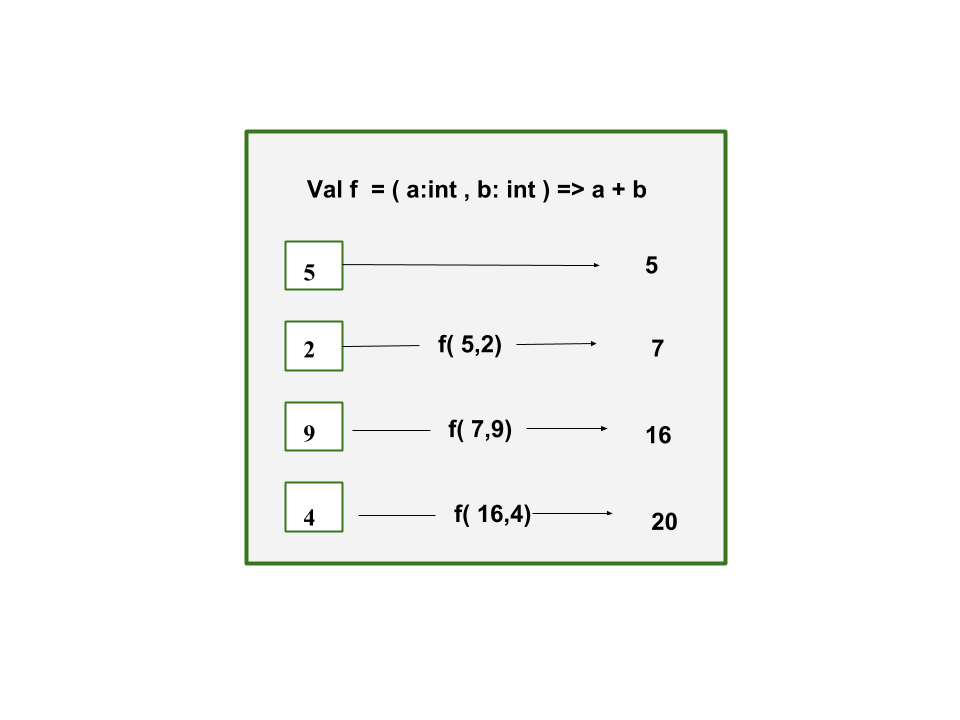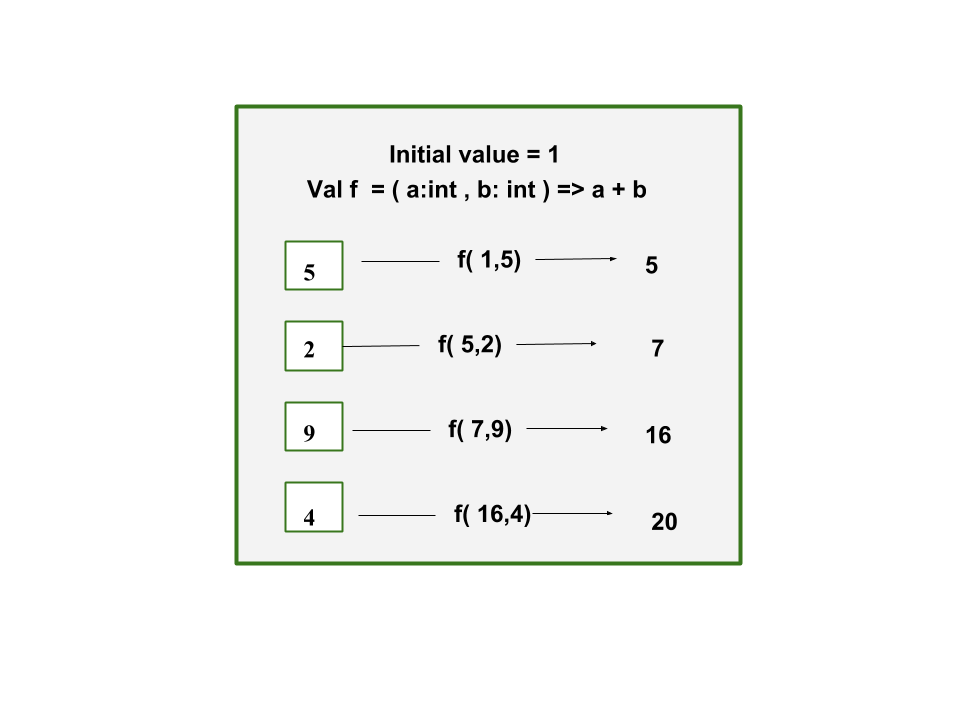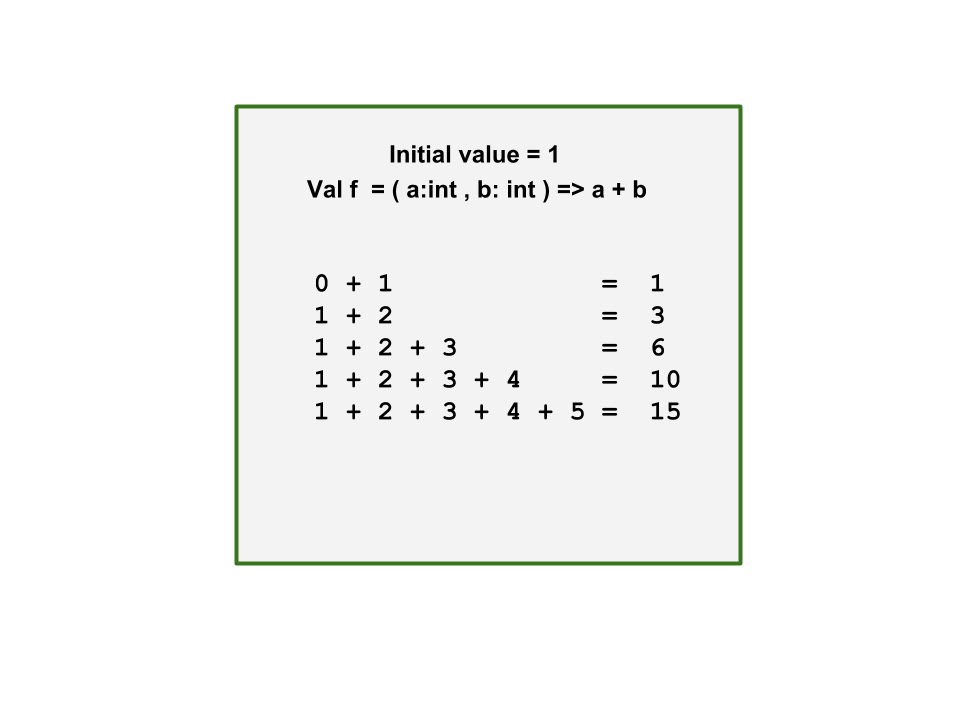# Scala | Reduce, fold or scan

In this tutorial we will learn about Reduce, Fold and Scan functions in Scala.

1. Reduce : Reduce function is applied on collection data structure in scala that contains lists, sets, maps, sequence and tuples. Parameter in the reduce function is a binary operation which merges all the elements from the collection and returns a single value. The first two values is combined with the binary operation and the resultant of that operation combines with the next value of the collection and atlast we obtain a single value.This code implements the Sum of elements in a sequence using reduce function.
Example :

 `// Scala program sum of elements  ` `// using reduce function  ` ` `  `// Creating object ` `object` `geeks ` `{ ` `    ``// Main method ` `    ``def` `main(arg``:``Array[String]) ` `    ``{ ` `        ``// initialize a sequence of elements ` `        ``val` `seq``_``elements``:` `Seq[Double] ``=` `Seq(``3.5``, ``5.0``, ``1.5``) ` `        ``println(s``"Elements = \$seq_elements"``)  ` ` `  `        ``// find the sum of the elements ` `        ``// using reduce function ` `        ``val` `sum``:` `Double ``=` `seq``_``elements.reduce((a, b) ``=>` `a + b) ` `        ``println(s``"Sum of elements = \$sum"``) ` `    ``}    ` `} `

Output:

```Elements  = List(3.5, 5.0, 1.5)
Sum of elements = 10.0
```

This code finds the maximum and minimum element in the sequence using reduce function
Example :

 `// Scala program to find maximum and minimum  ` `// using reduce function  ` ` `  `// Creating object ` `object` `geeks ` `{ ` `    ``// Main method ` `    ``def` `main(arg``:``Array[String]) ` `    ``{ ` `        ``// initialize a sequence of elements ` `        ``val` `seq``_``elements ``:` `Seq[Double] ``=` `Seq(``3.5``, ``5.0``, ``1.5``) ` `        ``println(s``"Elements = \$seq_elements"``) ` ` `  `        ``// find the maximum element using reduce function ` `        ``val` `maximum ``:` `Double ``=` `seq``_``elements.reduce(``_` `max ``_``) ` `        ``println(s``"Maximum element = \$maximum"``) ` ` `  `        ``// find the minimum element using reduce function ` `        ``val` `minimum ``:` `Double ``=` `seq``_``elements.reduce(``_` `min ``_``) ` `        ``println(s``"Minimum element = \$minimum"``) ` `    ``} ` `} `

Output:

```Elements = List(3.5, 5.0, 1.5)
Maximum element = 5.0
Minimum element = 1.5
```
2. Fold : Like reduce fold also takes a binary operation which merges all the elements from the collection and returns a single value. The difference is that fold allows us to define an initial value. Due to this property, fold can also manage empty collections. If the collection is empty, the value initialized becomes the final answer. Due to this we can also return a different value from the set of collection using initial value of some other datatype. Reduce can only return the value of the same type because its initial value is the first value from the collection.This code implements the Sum of elements in a sequence using fold function. Here initial value is taken as 0.0 as the sequence is in datatype Double.
Example :

 `// Scala program sum of elements  ` `// using fold function  ` ` `  `// Creating object ` `object` `geeks ` `{ ` `    ``// Main method ` `    ``def` `main(arg``:``Array[String]) ` `    ``{ ` `        ``// initialize a sequence of elements ` `        ``val` `seq``_``elements``:` `Seq[Double] ``=` `Seq(``3.5``, ``5.0``, ``1.5``) ` `        ``println(s``"Elements = \$seq_elements"``)  ` ` `  `        ``// find the sum of the elements using fold function ` `        ``val` `sum``:` `Double ``=` `seq``_``elements.fold(``0.0``)((a, b) ``=>` `a + b) ` `        ``println(s``"Sum of elements = \$sum"``) ` `    ``} ` `} `

Output:

```Elements = List(3.5, 5.0, 1.5)
Sum of elements = 10.0
```

This code concatenate the strings with hyphen. We use initial value as empty string. So our fold method will apply the operator on empty string as well where as with reduce we would not get the hyphen before the first value of the collection.
Example :

 `// Scala program concatenate string  ` `// using fold function  ` ` `  `// Creating object ` `object` `geeks ` `{ ` `    ``// Main method ` `    ``def` `main(arg``:``Array[String]) ` `    ``{ ` `        ``// initialize a sequence of strings ` `        ``val` `str``_``elements``:` `Seq[String] ``=` `Seq(``"hello"``, ` `                            ``"Geeks"``, ``"For"``, ``"Geeks"``) ` `        ``println(s``"Elements = \$str_elements"``)  ` ` `  `        ``// Concatenate strings with fold function ` `        ``val` `concat``:` `String ``=` `str``_``elements.fold(``""``)( ` `                                ``(a, b) ``=>` `a + ``"-"` `+ b) ` `        ``println(s``"After concatenation = \$concat"``) ` `    ``} ` `}     `

Output:

```Elements = List(hello, Geeks, For, Geeks)
After concatenation = -hello-Geeks-For-Geeks
```
3. Scan : Scan function takes the binary operation as parameter and returns the value for each element in collection for that operation. It returns each iteration for that binary operator in the collection. In scan also we can define the initial value.This code implements iterations of sum of all elements using scan function.
Example :

 `// Scala program sum of elements  ` `// using scan function  ` ` `  `// Creating object ` `object` `geeks ` `{ ` `    ``// Main method ` `    ``def` `main(arg``:``Array[String]) ` `    ``{ ` `        ``//initialize a sequence of numbers ` `        ``val` `numbers``:` `Seq[Int] ``=` `Seq(``4``, ``2``, ``1``, ``6``, ``9``) ` `        ``println(s``"Elements of numbers = \$numbers"``) ` ` `  `        ``//find the sum of the elements using scan function ` `        ``val` `iterations``:` `Seq[Int] ``=` `numbers.scan(``0``)(``_` `+ ``_``) ` `        ``println(``"Running total of all elements"` `+ ` `                ``s``"in the collection = \$iterations"``) ` `    ``} ` `}     `

Output:

```Elements of numbers = List(4, 2, 1, 6, 9)
Running total of all elements in the collection = List(0, 4, 6, 7, 13, 22)
```

This is the implementation of concatenation of the strings with hyphen and shows the iterations.
Example :

 `// Scala program concatenate string  ` `// using scan function  ` ` `  `// Creating object ` `object` `geeks ` `{ ` `    ``// Main method ` `    ``def` `main(arg``:``Array[String]) ` `    ``{ ` `        ``// initialize a sequence of strings ` `        ``val` `str``_``elements ``:` `Seq[String] ``=` `Seq(``"hello"``,  ` `                            ``"Geeks"``, ``"For"``, ``"Geeks"``) ` `        ``println(s``"Elements = \$str_elements"``) ` ` `  `        ``// Concatenate strings with scan function ` `        ``val` `concat ``:` `Seq[String] ` `                    ``=` `str``_``elements.scan(``""``)((a, b) ``=>` `a + ``"-"` `+ b) ` `        ``println(s``"After concatenation = \$concat"``) ` `    ``} ` `}     `

Output:

Elements = List(hello, Geeks, For, Geeks)
After concatenation = List(, -hello, -hello-Geeks, -hello-Geeks-For, -hello-Geeks-For-Geeks)

My Personal Notes arrow_drop_upCheck out this Author's contributed articles.

If you like GeeksforGeeks and would like to contribute, you can also write an article using contribute.geeksforgeeks.org or mail your article to contribute@geeksforgeeks.org. See your article appearing on the GeeksforGeeks main page and help other Geeks.

Please Improve this article if you find anything incorrect by clicking on the "Improve Article" button below.

Article Tags :

Be the First to upvote.

Please write to us at contribute@geeksforgeeks.org to report any issue with the above content.